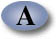UNIFIED ENGINEERING Fall 2004

Ian A. Waitz

Problem T5 (Unified Thermodynamics)

A thermally-insulated cylinder holds a thermally perfect gas at p = 4 atm and T = 300K. The gas is contained by a thermally-insulated massless piston with a stack of many small weights on top of it. The surroundings are at p = 1 atm and T = 300K. Initially the system is in mechanical and thermal equilibrium. Consider the following three processes:

a) All of the weights are removed from the piston instantaneously and the gas expands until the pressure matches that of the surroundings. How much work was done by the system? In the final state, what is the temperature and pressure of the system? Draw this process on a p-v diagram.

b) Half of the weight is removed from the piston instantaneously, the system is allowed to come to equilibrium, and then the remaining half of the weight is removed from the piston and the gas expands until the pressure matches that of the surroundings. How much work was done by the system? During the intermediate state and the final state, what is the temperature and pressure of the system? Draw this process on a p-v diagram.

c) Each small weight is removed from the piston one at a time, so that the pressure inside the cylinder can be assumed always to be in equilibrium with the weight on top of the piston. When the last weight is removed the gas has fully expanded to a pressure that matches that of the surroundings. How much work was done by the system? In the final state, what is the temperature and pressure of the system? Draw this process on a p-v diagram.

d) If your goal is to get as much work out of a system as possible without adding heat, what type of a process would you use?

Assume that cp = 1.0035 kJ/kg-K and cv = 0.7165 kJ/kg-K are constants, and that R = 0.287 kJ/kg-K.